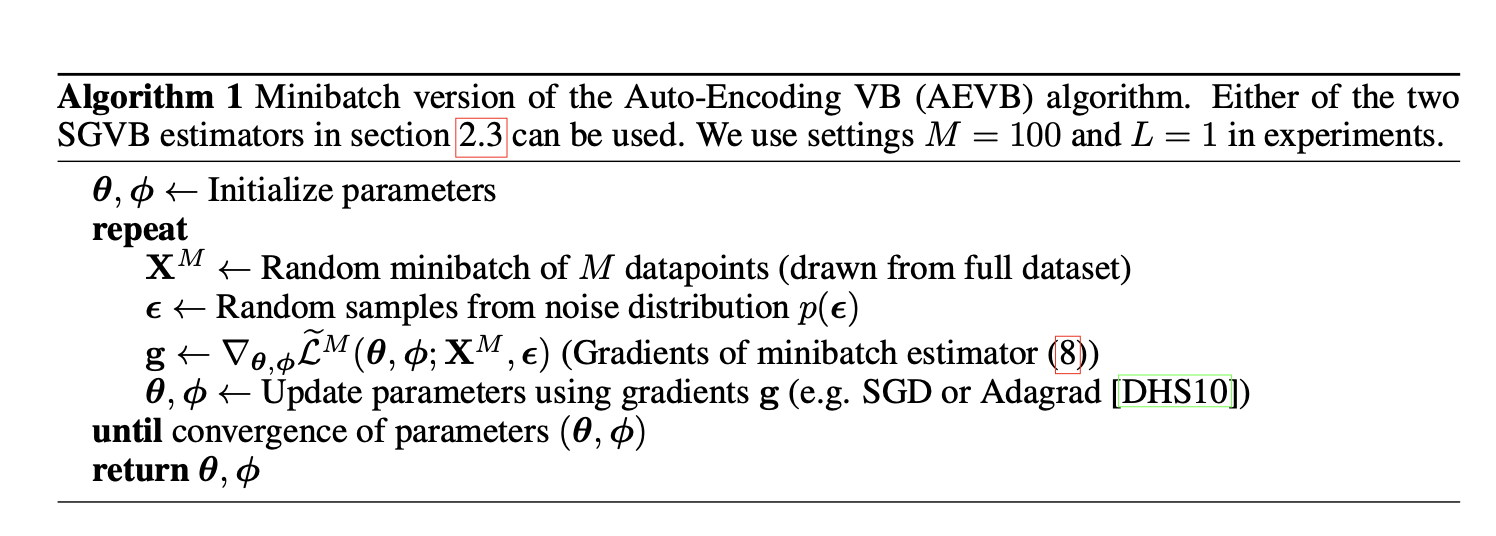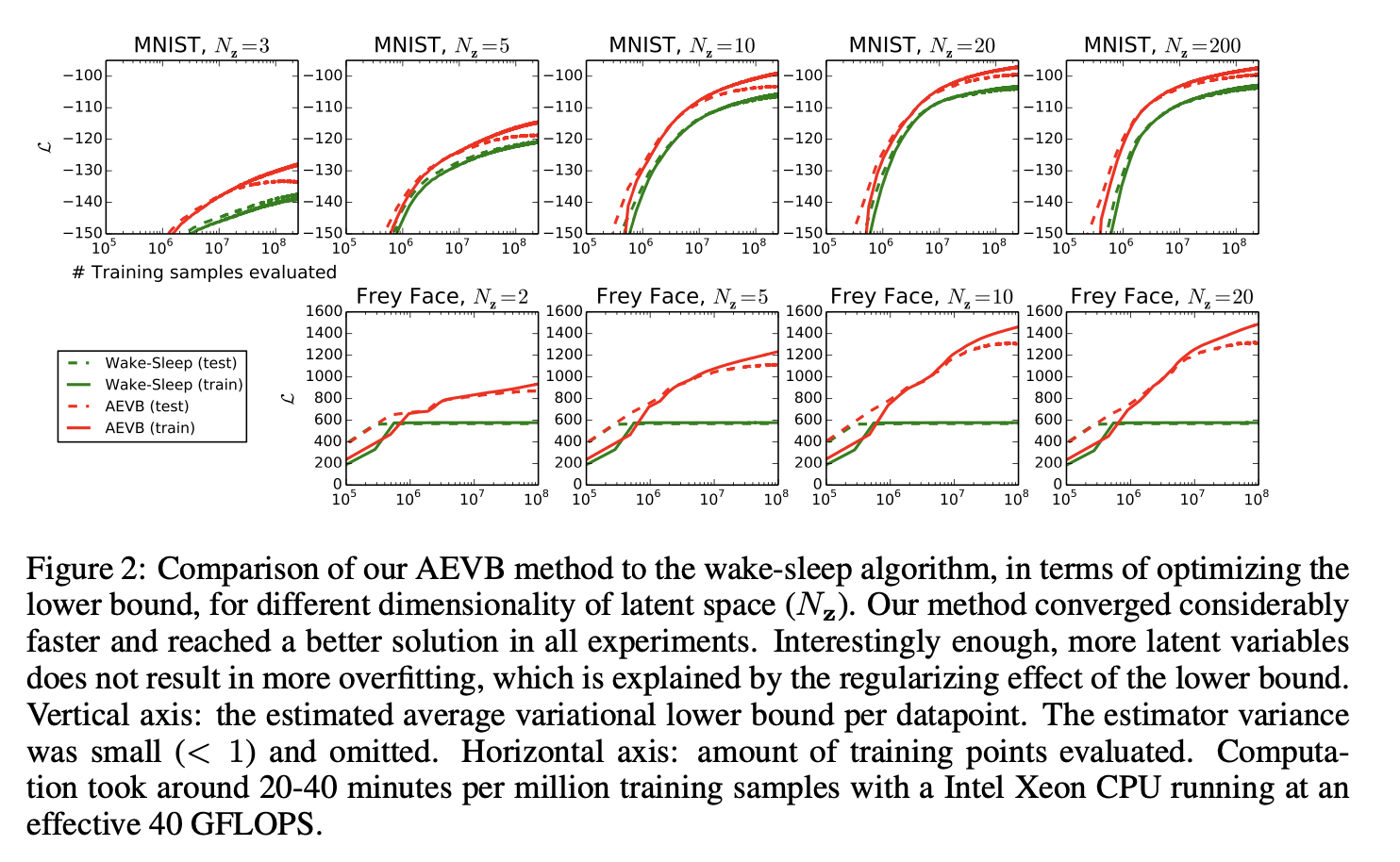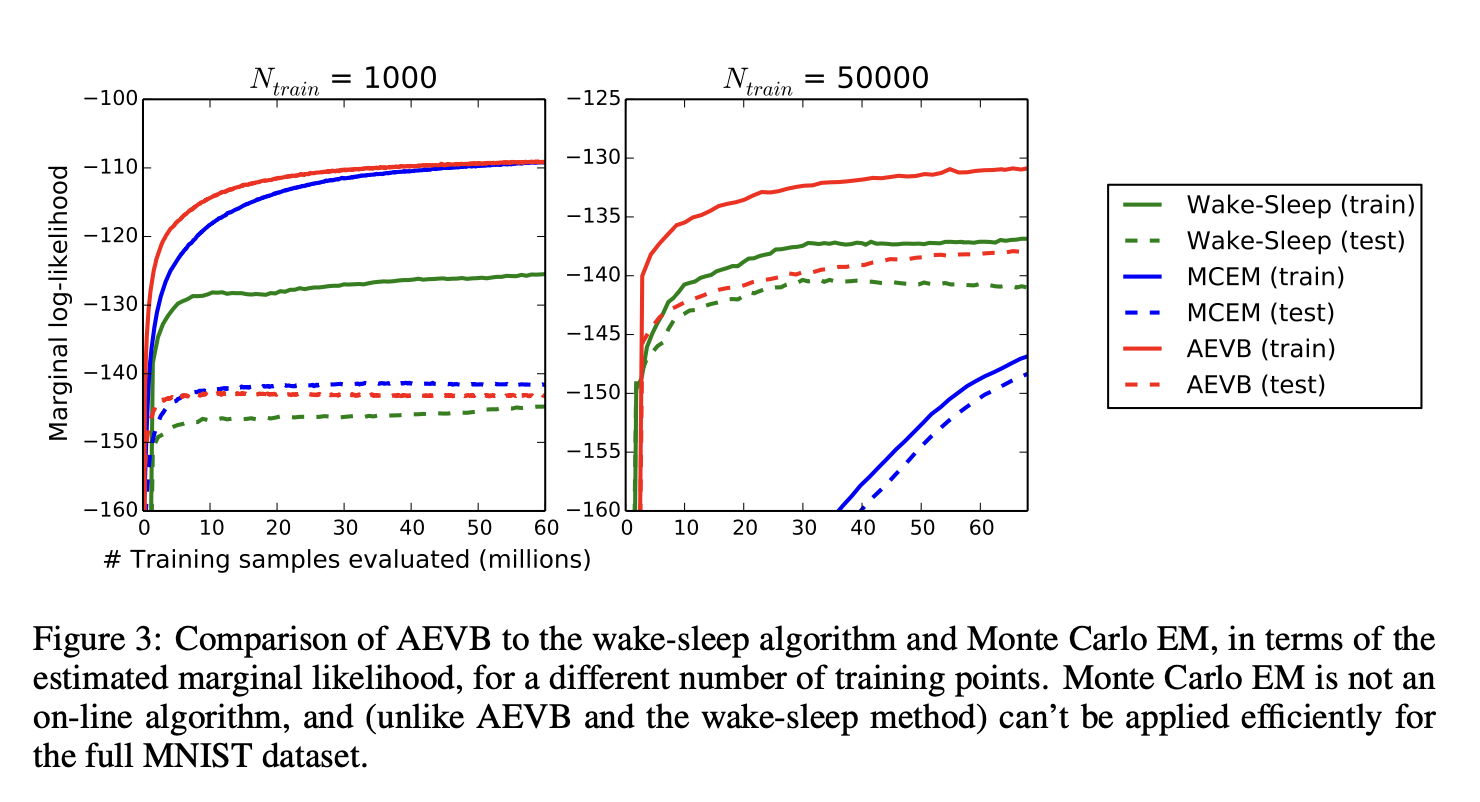# Auto-Encoding Variational Bayes

## Method

### Problem scenario• 从某个先验分布 $p_{θ^*}(z)$生成值 $z^{(i)}$；
• 从某个条件分布 $p_{θ^*}(x|z)$ 生成值 $x^{(i)}$。

1. 难处理性：边际似然 $p{\theta} = \int p{\theta}(z)p{\theta}(x|z)dz$ 的积分难以处理的情况（因此我们无法评估或区分边际似然），其中真实的后验密度 $p{\theta}(z|x) = p{\theta}(x|z)p{\theta}(z)/p{\theta}(x)$ 是难以处理的（因此不能使用 EM 算法），并且任何合理的平均场 VB 算法所需的积分也是难以处理的。这些难以处理的问题非常普遍，并且出现在中等复杂的似然函数 $p{\theta}(x|z)$ 的情况下，例如 具有非线性隐藏层的神经网络。
2. 大型数据集：我们有太多的数据，以至于批量优化成本太高； 我们希望使用小批量甚至单个数据点进行参数更新。 基于采样的解决方案，例如 Monte Carlo EM 通常会太慢，因为它涉及每个数据点通常昂贵的采样循环。

1. 参数 $θ$ 的有效近似 ML 或 MAP 估计。 参数本身可能是感兴趣的，例如 如果我们正在分析一些自然过程。 它们还允许我们模拟隐藏的随机过程并生成类似于真实数据的人工数据。
2. 给定观察值 $x$ 以选择参数 $θ$，对隐变量 $z$ 进行有效的近似后验推断。 这对于编码或数据表示任务很有用。
3. 变量 $x$ 的有效近似边际推断。 这使我们能够执行需要先验 $x$ 的各种推理任务。 计算机视觉中的常见应用包括图像去噪、修复和超分辨率。

### The SGVB estimator and AEVB algorithm### The reparameterization trick

1. 易处理的逆 CDF。 在这种情况下，令 $\epsilon \sim U(0,I)$ ，令 $g{\phi }(ε,x)$ 为 $q{\phi }(z|x)$ 的逆 CDF。 示例：指数、Cauchy、Logistic、Rayleigh、Pareto、Weibull、倒数、Gompertz、Gumbel 和 Erlang 分布。
2. 与高斯示例类似，对于任何“location-scale”分布族，我们可以选择标准分布（位置 = 0，尺度 = 1）作为辅助变量 $ε$，并令 $g(.) = local + scale·ε$ . 示例：拉普拉斯分布、椭圆分布、学生 t 分布、逻辑分布、均匀分布、三角分布和高斯分布。
3. 组合：通常可以将随机变量表示为辅助变量的不同变换。 示例：Log-Normal（正态分布变量的取幂）、Gamma（指数分布变量的总和）、Dirichlet（Gamma 变量的加权和）、Beta、卡方和 F 分布。

## Example: Variational Auto-Encoder

AEVB 算法揭示了有向概率模型（用变分目标训练）和自动编码器之间的联系。 线性自编码器和某类生成线性高斯模型之间的联系早已为人所知。 在 [Row98] 中表明，PCA 对应于线性高斯模型特例的最大似然 (ML) 解，其中先验 $p(z) = N (0, I)$ 和条件分布 $p(x |z) = N (x; Wz, \epsilon I)$，特别是 ε 无限小的情况。

## ExperimentsLikelihood lower bound 我们训练了生成模型（解码器）和相应的编码器（又名识别模型），在 MNIST 的情况下具有 500 个隐藏单元，在 Frey Face 数据集的情况下具有 200 个隐藏单元（以防止过度拟合，因为它是一个相当小的数据集）。 隐藏单元的选择数量是基于先前关于自编码器的文献，不同算法的相对性能对这些选择不是很敏感。 图 2 显示了比较下限时的结果。 有趣的是，多余的隐变量不会导致过拟合，这可以通过变分界的正则化性质来解释。

Marginal likelihood 对于非常低维的隐空间，可以使用 MCMC 估计器估计学习生成模型的边际似然。 有关边际似然估计量的更多信息，请参见附录。 对于编码器和解码器，我们再次使用了神经网络，这次有 100 个隐藏单元和 3 个隐变量； 对于更高维的潜在空间，估计变得不可靠。 同样，使用了 MNIST 数据集。 将 AEVB 和 Wake-Sleep 方法与具有混合蒙特卡罗 (HMC) [DKPR87] 采样器的蒙特卡罗 EM (MCEM) 进行了比较； 详细信息在附录中。 我们比较了三种算法的收敛速度，分别用于小型和大型训练集。 结果如图 3 所示。Visualisation of high-dimensional data 如果我们选择低维潜在空间（例如 2D），我们可以使用学习到的编码器（识别模型）将高维数据投影到低维流形。 有关 MNIST 和 Frey Face 数据集的 2D 潜在流形的可视化，请参见附录 A。

## Future work

• 使用用于编码器的深度神经网络（例如卷积网络）学习分层生成架构 和解码器，与 AEVB 联合训练；
• 时间序列模型（即动态贝叶斯网络）；
• 将 SGVB 应用于全局参数；
• 具有隐变量的监督模型，可用于学习复杂的噪声分布。